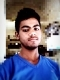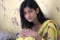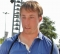## Fractional Knapsack ProblemUpdated on 15-Jun-2020 17:07:11
A list of items is given, each item has its own value and weight. Items can be placed in a knapsack whose maximum weight limit is W. The problem is to find the weight that is less than or equal to W, and value is maximized.There are two types of Knapsack problem.0 – 1 KnapsackFractional KnapsackFor the 0 – 1 Knapsack, items cannot be divided into smaller pieces, and for fractional knapsack, items can be broken into smaller pieces.Here we will discuss the fractional knapsack problem.The time complexity of this algorithm is O(n Log n).Input and OutputInput: Maximum weight = ... Read More

## Prim’s MST for Adjacency List RepresentationUpdated on 15-Jun-2020 17:13:06
It is similar to the previous algorithm. Here the only difference is, the Graph G(V, E) is represented by an adjacency list.Time complexity adjacency list representation is O(E log V).Input and OutputInput: The cost matrix: Output: Edge: A--B And Cost: 1 Edge: B--E And Cost: 2 Edge: A--C And Cost: 3 Edge: A--D And Cost: 4 Edge: E--F And Cost: 2 Edge: F--G And Cost: 3 Total Cost: 15Algorithmprims(g: Graph, start)Input −  The graph g and the seed vertex named ‘start’Output − The Tree after adding edges.Begin    create two set B, N    add the start node in B ... Read More

## Prim’s Minimum Spanning Tree AlgorithmUpdated on 15-Jun-2020 17:22:04
There is a connected graph G(V, E) and the weight or cost for every edge is given. Prim’s Algorithm will find the minimum spanning tree from the graph G. It is a growing tree approach. This algorithm needs a seed value to start the tree. The seed vertex is grown to form the whole tree.The problem will be solved using two sets. One set holds the nodes that are already selected, and another set holds the item those are not considered yet. From the seed vertex, it takes adjacent vertices, based on minimum edge cost, thus it grows the tree by ... Read More

## Minimum Number of Platforms ProblemUpdated on 15-Jun-2020 15:48:57
A list of arrival and departure time is given. Now the problem is to find the minimum number of platforms are required for the railway as no train waits.By sorting all timings in sorted order, we can find the solution easily, it will be easy to track when the train has arrived but not left the station.The time complexity of this problem is O(n Log n).Input and OutputInput: Lists of arrival time and departure time. Arrival: {900, 940, 950, 1100, 1500, 1800} Departure: {910, 1200, 1120, 1130, 1900, 2000} Output: Minimum Number of Platforms Required: 3AlgorithmminPlatform(arrival, departure, int n)Input − The ... Read More

## Minimum Coin Change ProblemUpdated on 15-Jun-2020 15:51:47
There is a list of coin C(c1, c2, ……Cn) is given and a value V is also given. Now the problem is to use the minimum number of coins to make the chance V.Note − Assume there are an infinite number of coins CIn this problem, we will consider a set of different coins C{1, 2, 5, 10} are given, There is an infinite number of coins of each type. To make change the requested value we will try to take the minimum number of coins of any type.As an example, for value 22 − we will choose {10, 10, 2}, ... Read More

## Kruskal’s Minimum Spanning Tree AlgorithmUpdated on 15-Jun-2020 16:00:50
There is a connected graph G(V, E) and the weight or cost for every edge is given. Kruskal’s algorithm will find the minimum spanning tree using the graph and the cost.It is the merge-tree approach. Initially, there are different trees, this algorithm will merge them by taking those edges whose cost is minimum, and form a single tree.In this problem, all of the edges are listed and sorted based on their cost. From the list, edges with minimum costs are taken out and added in the tree, and every there is a check whether the edge forming cycle or not, ... Read More

## Job Sequencing Problem with DeadlinesUpdated on 15-Jun-2020 16:04:43
In this problem, there is a list of jobs given. In the list, the deadline and the profits are also given for each job. Every job will take a single unit of time, so the minimum deadline for a job is 1. If only one job can be scheduled at a time, then maximize the profit.To solve this problem, all subset of the set of jobs are generated to check whether the individual subset is feasible or not. Also, keep track on maximum profit for all feasible subset that has generated.The time complexity of this algorithm is O(n^2)Input and OutputInput: ... Read More

## Efficient Huffman Coding for Sorted InputUpdated on 15-Jun-2020 16:08:20
In the previous Huffman code problem, the frequency was not sorted. If the frequency list is given in sorted order, the task of assigning code is being more efficient.In this problem, we will use two empty queues. Then create a leaf node for each unique character and insert it into the queue in increasing order of frequency.In this approach, the complexity of the algorithm is O(n).Input and OutputInput: Different letters and their frequency in sorted order Letters: {L, K, X, C, E, B, A, F} Frequency: {1, 1, 2, 2, 2, 2, 3, 4} Output: Codes for the letters L: ... Read More

## Huffman Coding AlgorithmUpdated on 23-Dec-2022 11:05:46
Huffman coding is a lossless data compression algorithm. In this algorithm, a variable-length code is assigned to input different characters. The code length is related to how frequently characters are used. Most frequent characters have the smallest codes and longer codes for least frequent characters.There are mainly two parts. First one to create a Huffman tree, and another one to traverse the tree to find codes.For an example, consider some strings “YYYZXXYYX”, the frequency of character Y is larger than X and the character Z has the least frequency. So the length of the code for Y is smaller than ... Read More

## Dijkstra’s Shortest Path AlgorithmUpdated on 15-Jun-2020 16:17:25
The main problem is the same as the previous one, from the starting node to any other node, find the smallest distances. In this problem, the main difference is that the graph is represented using the adjacency matrix. (Cost matrix and adjacency matrix is similar for this purpose).For the adjacency list representation, the time complexity is O(V^2) where V is the number of nodes in the graph G(V, E)Input and OutputInput: The adjacency matrix: Output: 0 to 1, Using: 0, Cost: 3 0 to 2, Using: 1, Cost: 5 0 to 3, Using: 1, Cost: 4 0 to 4, ... Read More